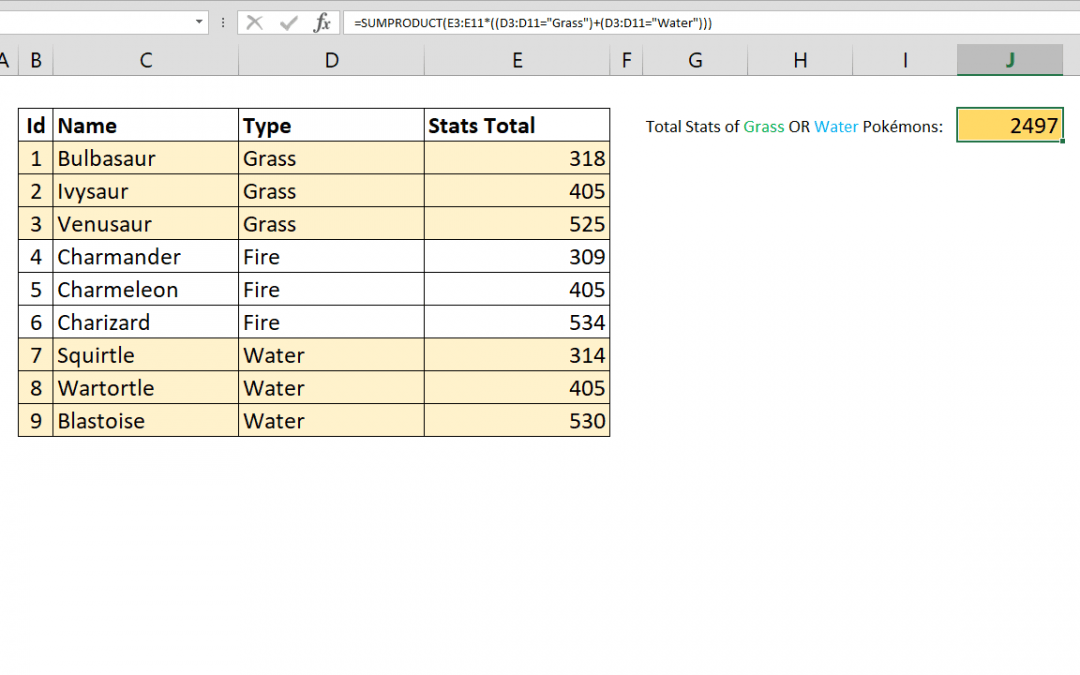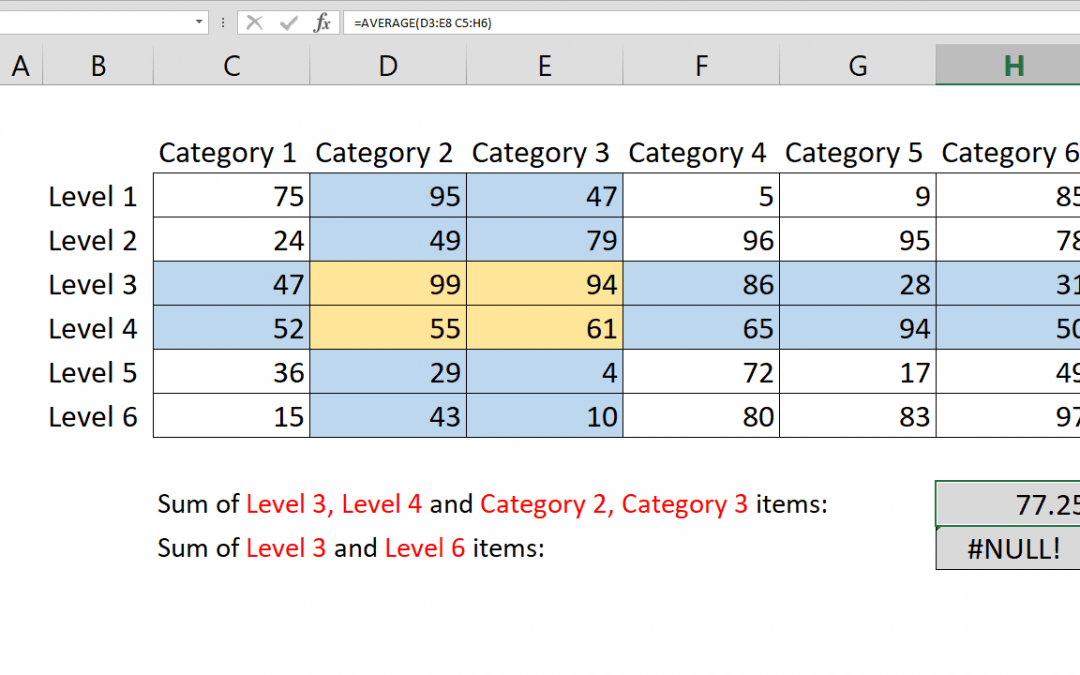## How to calculate running totals in Excel Tables

This article shows how to calculate running totals in Excel Tables by using structured references. If your data is not in a Table, please continue with this article: How to calculate running count Syntax =SUM(INDEX([column of values],1):[@ column of values]) Steps...## How to sum values with OR operator using SUMPRODUCT with multiple criteria

The SUMIFS function can sum values based on multiple conditions. However, these conditions are all linked with AND operator. As a result, all conditions should be fulfilled to return a value. This article shows How you can sum values with multiple OR operators using...## How to count characters in Excel in a range

The LEN function is used to count characters in Excel. But it doesn’t support ranges or arrays to easily count total number of characters in a range. This article shows 2 ways to count characters in Excel in a range which uses SUMPRODUCT and SUM along with LEN....## How to calculate SUM with intersecting tables

This article shows how to calculate sum with intersecting tables using SUM function and intersect operator, the space character (” “). Syntax =SUM(first range second range) Steps Type =SUM( function Select or type the first range reference that includes...## How to SUM 2d ranges with SUMPRODUCT

Summing values by a condition may sound easy. If not, please see Crunch Data Even Faster with the SUMIFS Function article as well. You can easily sum values by condition(s) with SUMIF and SUMIFS functions; with a limitation. The sum range and criteria ranges should...## How to SUM values across sheets

To sum range of cells in the same worksheet is one of the basic concepts of Excel. What about summing values across sheets? In this article we will explain how to sum values across sheets. Syntax =SUM(first sheet : second sheet ! reference) Steps Start with =SUM( Type...## How to sum filtered values in data tables

Excel’s regular SUM function includes all values in a range even if they are hidden by a filter or the right click Hide feature. This can be quite tedious when trying to create interactive spreadsheets that can utilize such controls. Using he SUBTOTAL function...